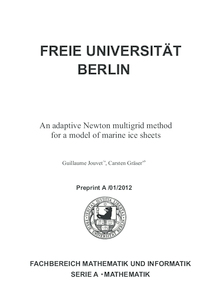Repository: Freie Universität Berlin, Math Department

# An adaptive Newton multigrid method for a model of marine ice sheets

Jouvet, G. and Gräser, C. (2013) An adaptive Newton multigrid method for a model of marine ice sheets. Journal of Computational Physics, 252 . pp. 419-437. ISSN 0021-9991Preview

1MB

Official URL: http://dx.doi.org/10.1016/j.jcp.2013.06.032

## Abstract

In this paper, we consider a model for the time evolution of three-dimensional marine ice sheets. This model combines the Shallow Ice Approximation (SIA) for the ice deformation, the Shallow Shelf Approximation (SSA) for the basal sliding, and the mass conservation principle. At each time step, we solve a scalar p-Laplace minimization-type problem with obstacle (SIA), a vectorial p-Laplace minimization-type problem (SSA) and a transport equation (mass conservation). The two minimization problems are solved using a truncated nonsmooth Newton multigrid method while the transport equation is solved using a vertex-centred finite volume method. Our approach is combined to an heuristic mesh adaptive refinement procedure to face the large gradients of the solution that are expected between the ice sheet and the ice shelf. As applications, we present some simulations of the Marine Ice Sheet Model Intercomparison Project MISMIP (2D and 3D) and validate our results against an analytic solution (2D) and other participant model results (3D). Further numerical results show that the convergence of our Newton multigrid method is insensitive to local refinements making our overall adaptive strategy fully efficient.

Item Type: Article Marine ice sheets, Grounding line, p-Laplace, Newton multigrid method, Adaptive method Mathematical and Computer Sciences > Mathematics > Numerical Analysis Department of Mathematics and Computer Science > Institute of Mathematics 1794 Ekaterina Engel 17 Feb 2016 10:01 03 Mar 2017 14:41

Repository Staff Only: item control page